# OECD Adult education level data analysis 9 - regression analysis with panel data using RUnsplashMartin Sanchezが撮影した写真

This post is following of the above post. In the previous post, I made a panel data frame, n = 37, T = 2, N = 74. In this post, I do regression analysis with the panel data frame.

First, I analyze pooled cross sectiona regression, I mean I just use the panel data as simple pooled cross section data.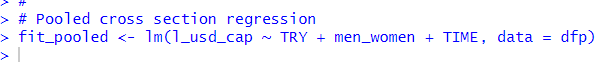Let's see the results with summary() function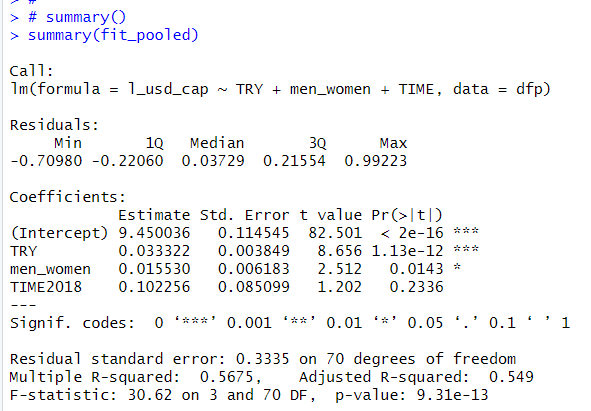Whole p-value is almost 0, TRY estimate is 0.033 and p-value is almost 0. men_women estimate is 0.016 and p-value is 0.014. So, TRY and men_woemn have the positive effect to l_usd_cap and the both are statistically significant. TIME2018 is not statistically significant.

Next, let's do panel data regression.

In my mind, I have following model formula.

l_usd_cap = beta0 + beta1 * TRY + beta2 *  men_woemen + beta3 * TIME2018 + a + u.

a is a LOCATION specific effect to l_usd_cap and it is the same in 2008 and 2018.

u is an error term.

Since a is not observed, I want to remove a. To remove a, I use folloing two formula,

For 2008,

l_usd_cap(2008) = beta0 + beta1 * TRY(2008) + beta2 * men_women(2008) + beta3 * 0 + a + u,

For 2018,

l_usd_cap(2018) = beta0 + beta1 * TRY(2018) + beta2 * men_women(2008) + beta3 * 1 + a + u

Formula 2018 - Formula 2008 makes

l_usd_cap(2018) - l_usd_cap(2008) = beta1 * (TRY(2018) - TRY(2008)) + beta2 * (men_women(2018) - men_women(2008)) + beta3 * (1 - 0) + u.

Now, a is removed.

This is first-differenced estimator.

I can do it with plm() frunction with plm package.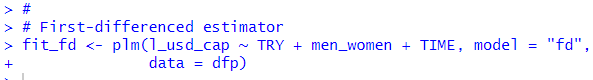Let's see the result with summary() function.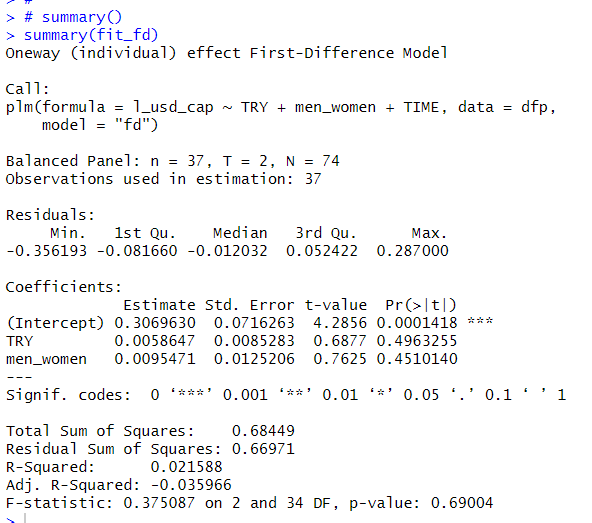I see with first differenced estimator, TRY and men_women is not associated with l_usd_cap.

With plm() function, I can do pooling cross section regression with model = "pooling".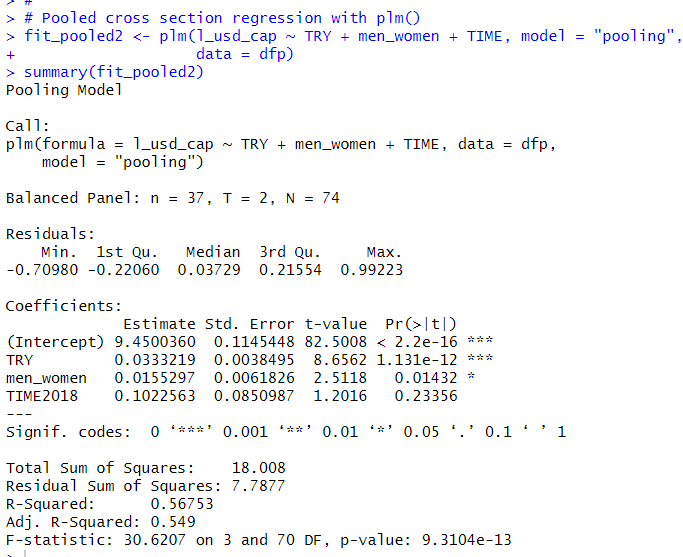This is the same results as fit_pooled.
That's it. Thank you!

To read from the 1st post,